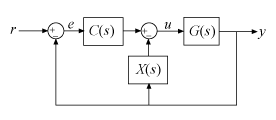getComponents

Extract SISO control components from a 2-DOF PID controller

Description

example

[C,X] = getComponents(C2,looptype) decomposes the 2-DOF PID controller C2 into two SISO control components. One of the control components, C, is a 1-DOF PID controller. The other, X, is a SISO dynamic system. When C and X are connected in the loop structure specified by looptype, the resulting closed-loop system is equivalent to the 2-DOF control loop.

Examples

collapse all

Decompose a 2-DOF PID controller into SISO control components, using each of the feedforward, feedback, and filter configurations.

To start, obtain a 2-DOF PID controller. For this example, create a plant model and tune a 2-DOF PID controller for it.

G = tf(1,[1 0.5 0.1]);
C2 = pidtune(G,'pidf2',1.5)
C2 =

1                s
u = Kp (b*r-y) + Ki --- (r-y) + Kd -------- (c*r-y)
s              Tf*s+1

with Kp = 1.12, Ki = 0.23, Kd = 1.3, Tf = 0.122, b = 0.664, c = 0.0136

Continuous-time 2-DOF PIDF controller in parallel form.

C2 is a pid2 controller object, with two inputs and one output. Decompose C2 into SISO control components using the feedforward configuration.

[Cff,Xff] = getComponents(C2,'feedforward')
Cff =

1            s
Kp + Ki * --- + Kd * --------
s          Tf*s+1

with Kp = 1.12, Ki = 0.23, Kd = 1.3, Tf = 0.122

Continuous-time PIDF controller in parallel form.

Xff =

-10.898 (s+0.2838)
------------------
(s+8.181)

Continuous-time zero/pole/gain model.

As the display shows, this command returns the SISO PID controller Cff as a pid object. The feedforward compensator X is returned as a zpk object.

Decompose C2 using the feedback configuration. In this case as well, Cfb is a pid controller object, and the feedback compensator X is a zpk model.

[Cfb,Xfb] = getComponents(C2,'feedback');

Decompose C2 using the filter configuration. Again, the components are a SISO pid controller and a zpk model representing the prefilter.

[Cfr,Xfr] = getComponents(C2,'filter');

Input Arguments

collapse all

2-DOF PID controller to decompose, specified as a pid2 or pidstd2 controller object.

Loop structure for decomposing the 2-DOF controller, specified as 'feedforward', 'feedback', or 'filter'. These correspond to the following control decompositions and architectures:

• 'feedforward'C is a conventional SISO PID controller that takes the error signal as its input. X is a feedforward controller, as shown:If C2 is a continuous-time, parallel-form controller, then the components are given by:

$\begin{array}{c}C\left(s\right)={K}_{p}+\frac{{K}_{i}}{s}+\frac{{K}_{d}s}{{T}_{f}s+1},\\ X\left(s\right)=\left(b-1\right){K}_{p}+\frac{\left(c-1\right){K}_{d}s}{{T}_{f}s+1}.\end{array}$

The following command constructs the closed-loop system from r to y for the feedforward configuration.

T = G*(C+X)*feedback(1,G*C);
• 'feedback'C is a conventional SISO PID controller that takes the error signal as its input. X is a feedback controller from y to u, as shown:If C2 is a continuous-time, parallel-form controller, then the components are given by:

$\begin{array}{c}C\left(s\right)=b{K}_{p}+\frac{{K}_{i}}{s}+\frac{c{K}_{d}s}{{T}_{f}s+1},\\ X\left(s\right)=\left(1-b\right){K}_{p}+\frac{\left(1-c\right){K}_{d}s}{{T}_{f}s+1}.\end{array}$

The following command constructs the closed-loop system from r to y for the feedback configuration.

T = G*C*feedback(1,G*(C+X));
• 'filter'X is a prefilter on the reference signal. C is a conventional SISO PID controller that takes as its input the difference between the filtered reference and the output, as shown:If C2 is a continuous-time, parallel-form controller, then the components are given by:

$\begin{array}{c}C\left(s\right)={K}_{p}+\frac{{K}_{i}}{s}+\frac{{K}_{d}s}{{T}_{f}s+1},\\ X\left(s\right)=\frac{\left(b{K}_{p}{T}_{f}+c{K}_{d}\right){s}^{2}+\left(b{K}_{p}+{K}_{i}{T}_{f}\right)s+{K}_{i}}{\left({K}_{p}{T}_{f}+{K}_{d}\right){s}^{2}+\left({K}_{p}+{K}_{i}{T}_{f}\right)s+{K}_{i}}.\end{array}$

The following command constructs the closed-loop system from r to y for the filter configuration.

T = X*feedback(G*C,1);

The formulas shown above pertain to continuous-time, parallel-form controllers. Standard-form controllers and controllers in discrete time can be decomposed into analogous configurations. The getComponents command works on all 2-DOF PID controller objects.

Output Arguments

collapse all

SISO PID controller, returned as a pid or pidstd controller object. The form of C corresponds to the form of the input controller C2. For example, if C2 is a standard-form pidstd2 controller, then C is a pidstd object.

The precise functional form of C depends on the loop structure you specify with the looptype argument, as described in Input Arguments.

SISO control component, specified as a zero-pole-gain (zpk) model. The precise functional form of X depends on the loop structure you specify with the looptype argument, as described in Input Arguments.